# Applying rpatrec to real-life data

The package comes equipped with a large amount of stock market data for testing purposes. The data is obtained from Datastream. The file contains the daily closing prices of the DAX30, Dow Jones Industrials and the Nikkei 225.

This vignette is not thought to be stand alone documentation but should be read with the report published at the same time.

First, plot the DJI (with patterns marked between colourful lines) and plot the sections that the human observer recognises as patterns. This is our benchmark for this exploration.

dji <- sample.pre(data[])
#>  "cut input by" "259"

plot(dji,type="l",main= "Dow Jones Industrials",xlab="Trading Days",ylab="Closing Price",col="blue")
abline(v=400, col="red")
abline(v=1250,col="red")

abline(v=1500, col="green")
abline(v=2100,col="green")

abline(v=2300, col="yellow")
abline(v=2800,col="yellow")

plot(dji[400:1250],type="l",main= "Inverse HS",xlab="Trading Days",ylab="Closing Price",col="blue")
plot(dji[1500:2100],type="l",main= "Dow Jones Industrials",xlab="Trading Days",ylab="Closing Price",col="blue")
plot(dji[2300:2800],type="l",main= "Dow Jones Industrials",xlab="Trading Days",ylab="Closing Price",col="blue")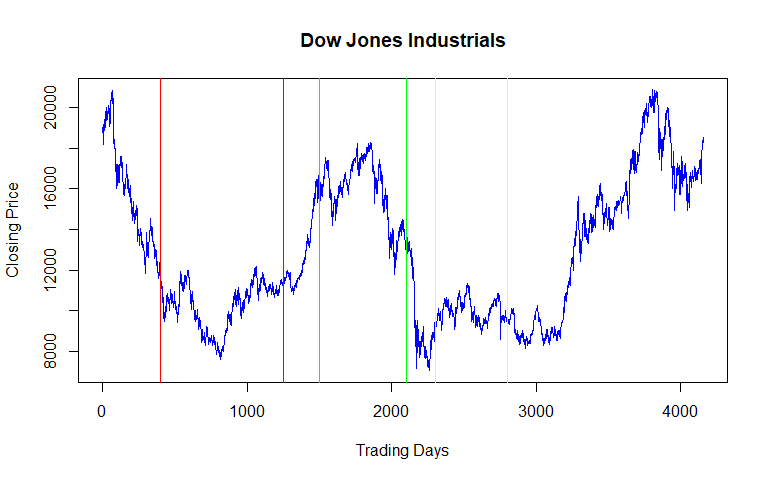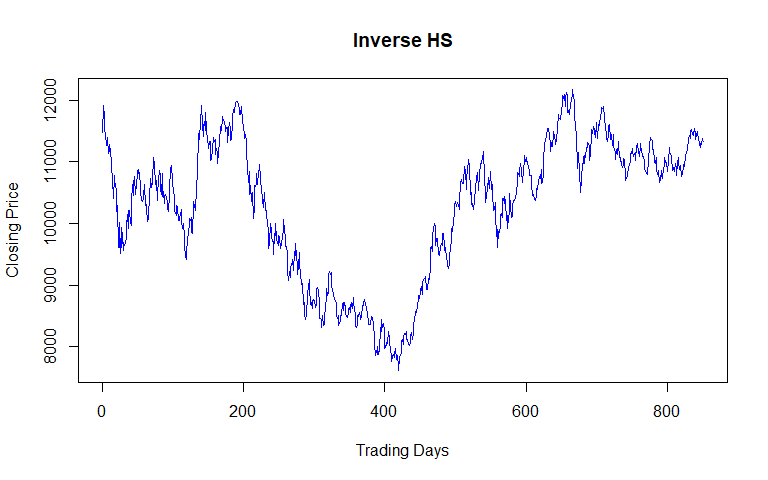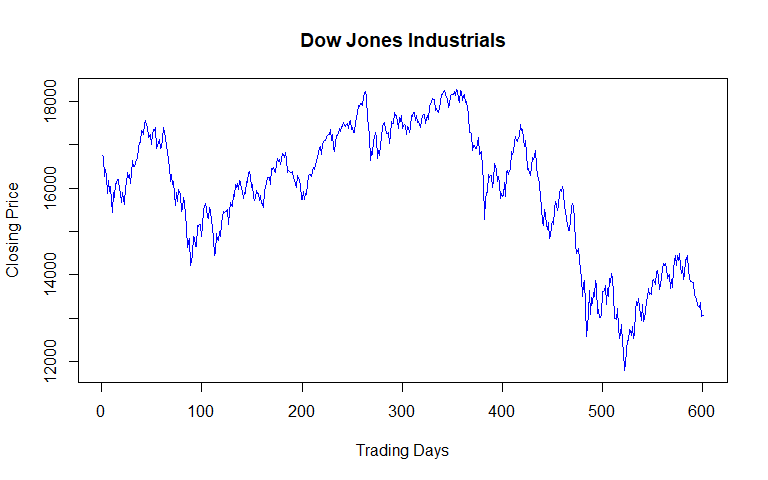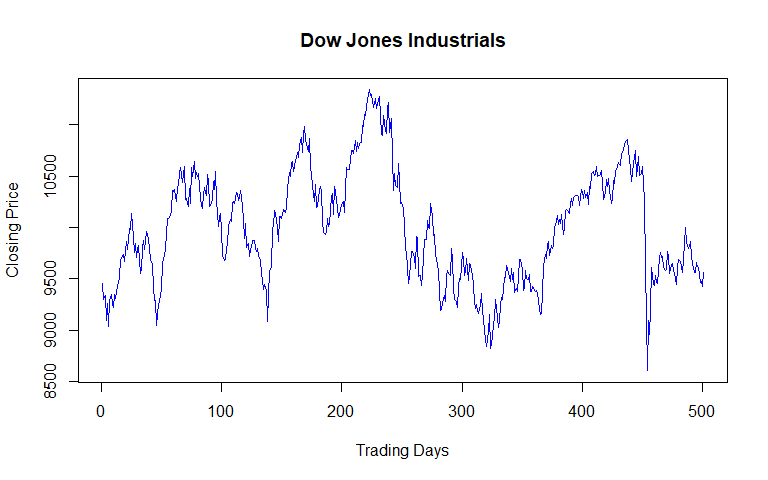Now see whether the algorithms can do the same: First, the data is smoothed: We are looking for long-term pattern, so we set the smoother to a high value: Plot again with patterns still highlighted from before


a <- kernel(dji,30)
plot(a,type="l",main= "Dow Jones Industrials",xlab="Trading Days",ylab="Closing Price",col="blue")
abline(v=400, col="red")
abline(v=1250,col="red")

abline(v=1500, col="green")
abline(v=2100,col="green")

abline(v=2300, col="yellow")
abline(v=2800,col="yellow")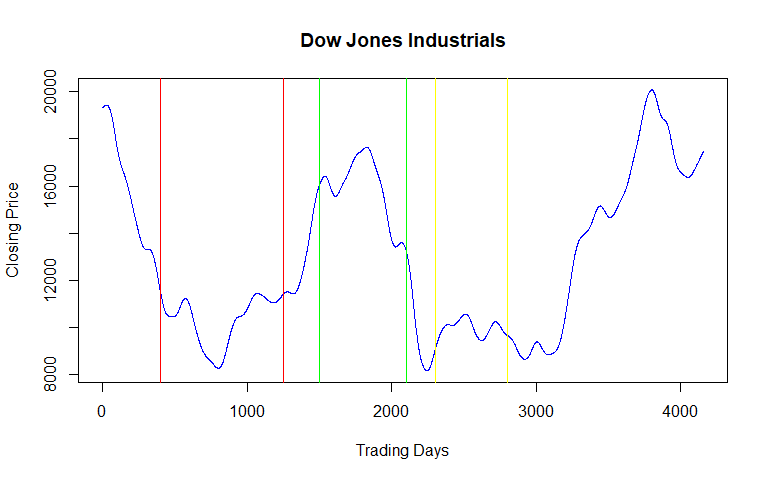Now, run it through the slicer function to see what the package makes of it:


slicer(a,750,150,btpiq=FALSE,rtpiq=FALSE,dtpiq=FALSE)
#>  "Patterns were found in "
#>  "29"
#>  "% of windows analysed. Refer to function value for details"
#> []
#>   0 0 0 1 0 0 0 0 0 1 1 0 0 0 1 1 1 1 0 0 0 0 0 0
#>
#> []
#>   450 1350 1500 2100 2250 2400 2550
plot(a[450:1200],type="l",main= "Dow Jones Industrials",xlab="Trading Days",ylab="Closing Price",col="blue")
plot(a[1350:2350],type="l",main= "Dow Jones Industrials",xlab="Trading Days",ylab="Closing Price",col="blue")
plot(a[2100:3300],type="l",main= "Different windows",xlab="Trading Days",ylab="Closing Price",col="blue")

abline(v=0)
abline(v=750)

abline(v=150, col="red")
abline(v=900, col="red")

abline(v=300, col="green")
abline(v=1050, col="green")

abline(v=450,col = "yellow")
abline(v=1200,col = "yellow")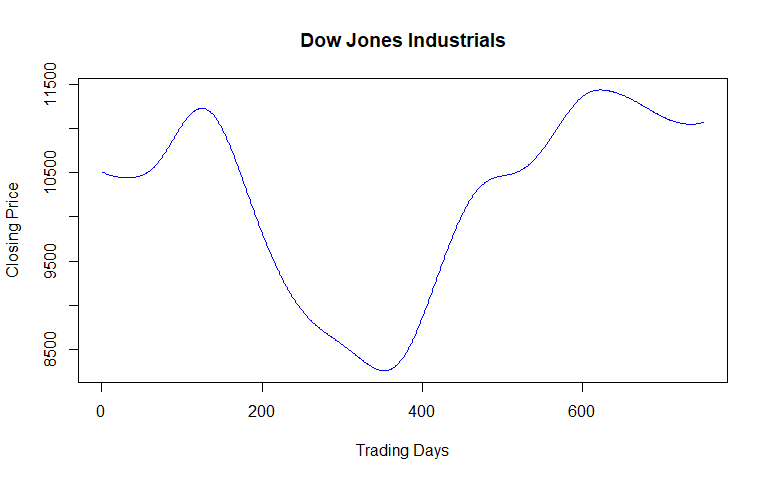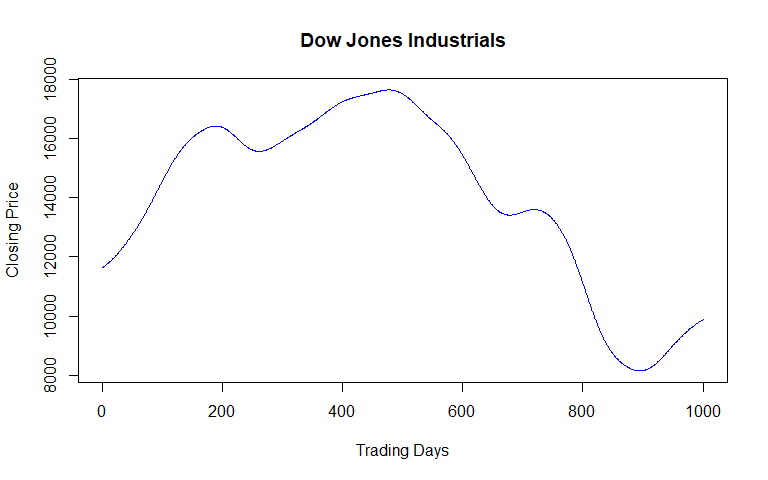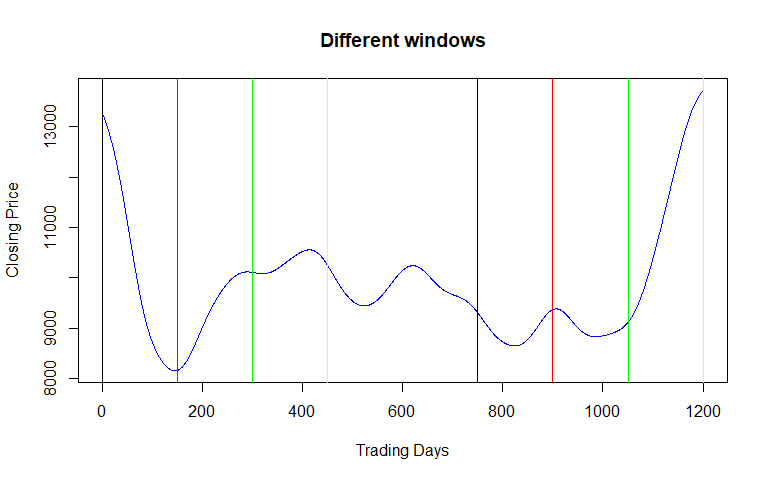interpret(a[2100:2850])
#> $EXT #>  0 1 0 1 0 1 #> #>$EXV
#>   8155.584 10117.672 10082.070 10555.243  9442.936 10240.981
#>
#> $EXP #>  145 290 320 413 523 620 #> #>$HSP
#> $HSP$HS
#>  10117.672 10082.070 10555.243  9442.936 10240.981
#>
#>
#> $BTPorTTP #>  NA #> #>$RTP
#>  NA
#>
#> $DTP #>$DTP$DTOP #>  10117.67 10082.07 10555.24 #> #> #>$RESULT
#>  TRUE
interpret(a[2250:3000])
#> $EXT #>  1 0 1 0 1 0 #> #>$EXV
#>  10117.672 10082.070 10555.243  9442.936 10240.981  8649.195
#>
#> $EXP #>  140 170 263 373 470 673 #> #>$HSP
#> $HSP$HS
#>  10117.672 10082.070 10555.243  9442.936 10240.981
#>
#>
#> $BTPorTTP #>  NA #> #>$RTP
#>  NA
#>
#> $DTP #>$DTP$DTOP #>  10117.67 10082.07 10555.24 #> #> #>$RESULT
#>  TRUE

For explanation of these results refer to the report.